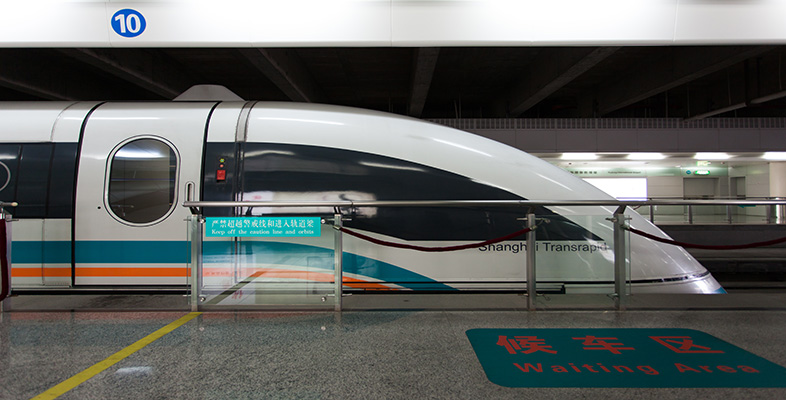Superconductivity

Start this free course now. Just create an account and sign in. Enrol and complete the course for a free statement of participation or digital badge if available.

Free course

# 2 Properties of superconductors

## 2.1 Zero electrical resistance

In this section we shall discuss some of the most important electrical properties of superconductors, with discussion of magnetic properties to follow in the next section.

The most obvious characteristic of a superconductor is the complete disappearance of its electrical resistance below a temperature that is known as its critical temperature. Experiments have been carried out to attempt to detect whether there is any small residual resistance in the superconducting state. A sensitive test is to start a current flowing round a superconducting ring and observe whether the current decays. The current flowing in the superconducting loop clearly cannot be measured by inserting an ammeter into the loop, since this would introduce a resistance and the current would rapidly decay.

### SAQ 1

Suggest a method of monitoring the current that does not involve interfering with the superconducting loop.

The magnetic field generated by the current in the loop could be monitored.

The magnitude of the magnetic field is directly proportional to the current circulating in the loop, and the field can be measured without drawing energy from the circuit. Experiments of this type have been carried out over periods of years, and the magnetic field – and hence the superconducting current – has always remained constant within the precision of the measuring equipment. Such a persistent current is characteristic of the superconducting state. From the lack of any decay of the current it has been deduced that the resistivity ρ of a superconductor is less than 10﻿−﻿26 Ω m. This is about 18 orders of magnitude smaller than the resistivity of copper at room temperature (10﻿−﻿8 Ω m﻿).

Resistivity is the reciprocal of conductivity, that is, ρ = σ﻿﻿−﻿1. We prefer to describe a superconductor by ρ = 0﻿, rather than by σ =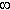.

In the following Exercise you can estimate an upper limit for the resistivity of a superconductor.

### Exercise 1

(a) A circuit, with self-inductance L, has a current I﻿0 flowing in it at time t = 0. Assuming that the circuit has a small residual resistance R but contains no source of emf, what will be the current in the circuit after a time T has elapsed?

(b) In a classic experiment performed by Quinn and Ittner in 1962, a current was set up around the ‘squashed tube’, shown in Figure 5, which was made from two thin films of superconducting lead separated by a thin layer of insulating silicon oxide. The inductance L of the tube was estimated to be 1.4 × 10﻿−﻿13 H. No change in the magnetic moment due to the current could be detected after 7 hours, to within the 2 per cent precision of their measurement, so the current was at least 98 per cent of its initial value. Estimate the maximum possible resistance of the tube for circulating currents.

(c) The dimensions of the tube used in the experiment are shown in Figure 5. Estimate the maximum possible resistivity ρmax of the lead films. Compare your answer with the resistivity of pure lead at 0°C, which is 1.9  ×  10﻿–﻿7﻿Ω m.

(a) The current in an RL circuit (with no source of emf) decays exponentially with time: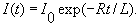The time constant for the decay is L/R, so the smaller the resistance, the longer will be the time constant. Zero resistance means an infinite time constant – the current does not decay, but persists indefinitely (or as long as the material remains superconducting).

(b) The maximum possible resistance, Rmax, of the tube corresponds to the minimum possible current, which is I(T) = 0.98I0. Thus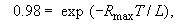which can be rearranged to give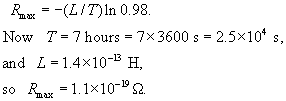(c) For the tube, the length of the current path,I, is essentially twice the width of the silicon oxide layer, and the cross-sectional area perpendicular to current flow, A﻿I, is the length of the tube times the thickness of the lead films. Since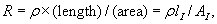the maximum resistivity is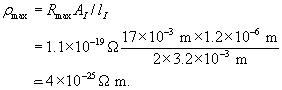The value of the resistivity of lead at 0°C is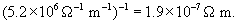The estimate for the maximum resistivity for superconducting lead obtained from the data in this exercise is almost 18 orders of magnitude smaller than this room temperature value.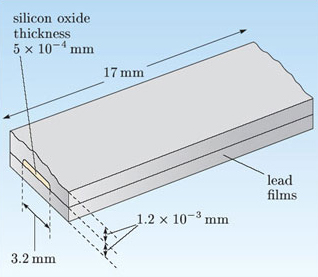Figure 5 A lead ‘tube’ used in a persistent current experiment.

SMT359_1